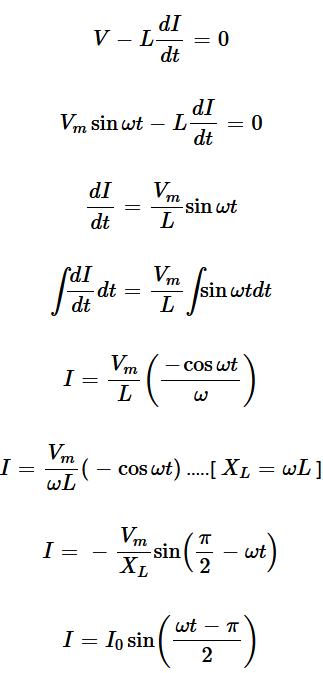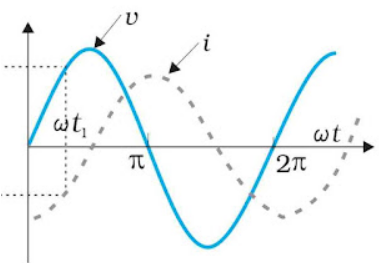# Alternating Current Circuits

### Alternating Current (A.C.) Source

An alternating current changes its direction of flow periodically. For a half cycle, it flows in one direction and for the next half cycle, it flows in opposite direction.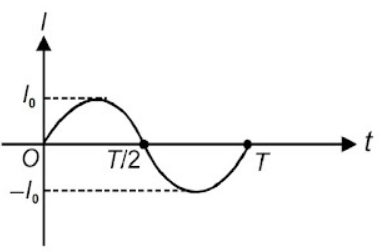### Mean value for half-cycle of AC

Mean value of AC is the total charge that flows through a circuit element in a given time interval divided by the time interval.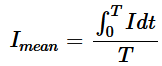For half cycle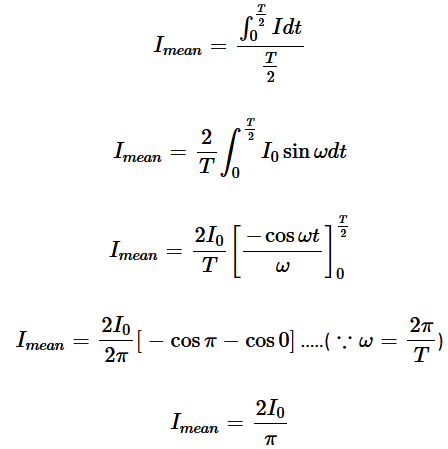Note :  For complete cycle, mean value = 0

RMS value of alternating supply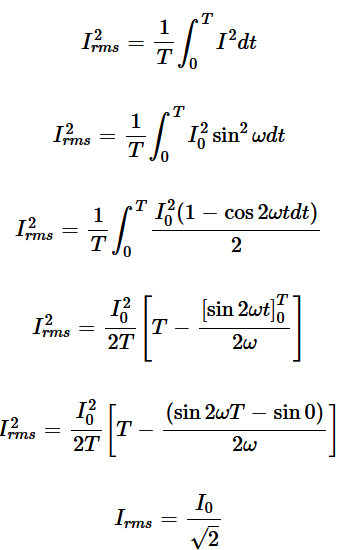### A.C. Voltage Applied to a Resistor

V = Vmsinωt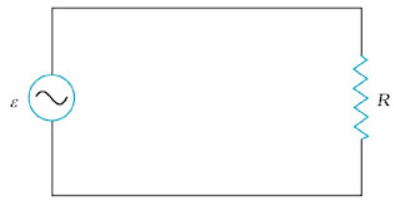The instantaneous power dissipated in the resistor is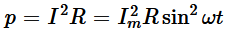The average value of ‘p’ over a cycle is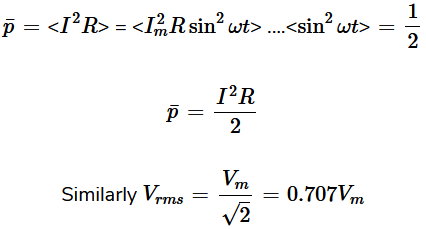To find the value of current through the resistor, we apply ohm's law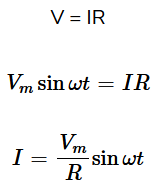Since R is a constant, we can write this equation as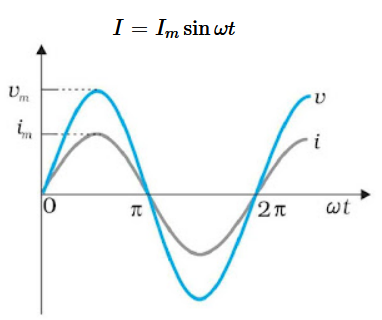In a pure resistor, the voltage and current are in phase. The minima, zero, and maxima occur at the same respective times.

Alternating Voltage Applied to an Inductor
Let the voltage across the source be V = Vm sinωt.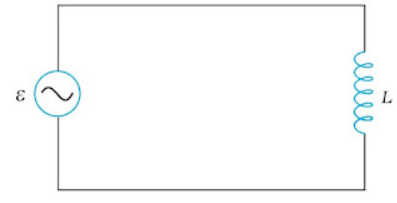If, there be no resistor in the circuit,